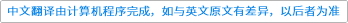Communications in Contemporary Mathematics ( IF 1.278 ) Pub Date : 2020-12-29 , DOI: 10.1142/s0219199720500923
Grzegorz Pastuszak; Adam Skowyrski; Andrzej Jamiołkowski

Assume that $Φ:𝕄n(ℂ)→𝕄n(ℂ)$ is a superoperator which preserves hermiticity. We give an algorithm determining whether $Φ$ preserves semipositivity (we call $Φ$positive in this case). Our approach to the problem has a model-theoretic nature, namely, we apply techniques of quantifier elimination theory for real numbers. An approach based on these techniques seems to be the only one that allows to decide whether an arbitrary hermiticity-preserving $Φ$ is positive. Before we go to detailed analysis of the problem, we argue that quantifier elimination for real numbers (and also for complex numbers) can play a significant role in quantum information theory and other areas as well.down
wechat
bug